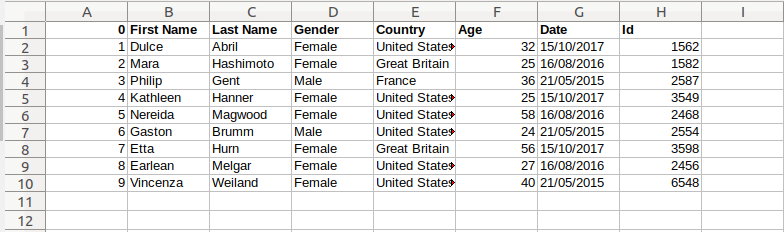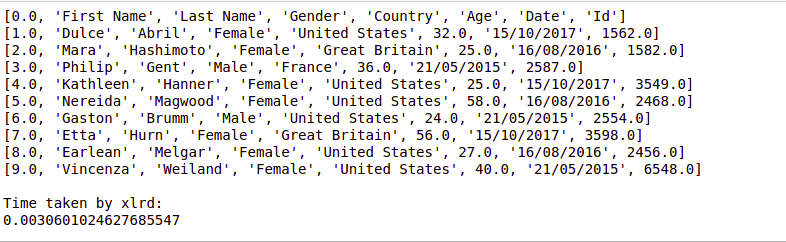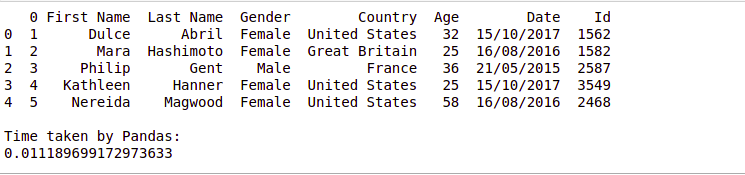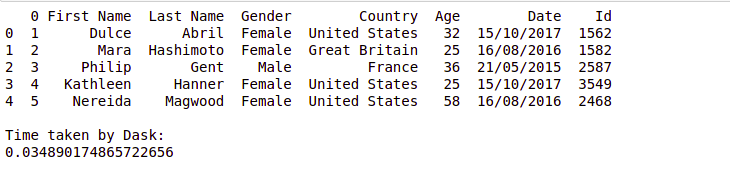# Data Ingestion via Excel: Comparing runtimes

Data ingestion is the process of obtaining and importing the data for the storage in the database. In this article, we explore different data ingestion techniques used to extract the data from the excel file in Python and compare their runtimes.

Let’s suppose the excel file looks like this –#### Using xlrd library

Using `xlrd` module, one can retrieve information from a spreadsheet. For example, reading, writing or modifying the data can be done in Python. Also, user might have to go through various sheets and retrieve data based on some criteria or modify some rows and columns and do a lot of work.

 `import` `xlrd ` `import` `time ` ` `  ` `  `# Time variable for finding the  ` `# difference ` `t1 ``=` `time.time() ` ` `  `#Open the workbook to read the ` `# excel file  ` `workbook ``=` `xlrd.open_workbook(``'excel.xls'``) ` `  `  `#Get the first sheet in the workbook  ` `sheet ``=` `workbook.sheet_by_index(``0``) ` ` `  `#Read row data line by line  ` `for` `i ``in` `range``(sheet.nrows): ` `    ``row ``=` `sheet.row_values(i)  ` `    ``print``(row) ` `     `  `t2 ``=` `time.time() ` `print``(``"\nTime taken by xlrd:"``) ` `print``(t2``-``t1) `

Output:#### Using Pandas

Python data analysis library is a powerful tool used by data scientists. It helps in data ingestion and data exploration.

 `import` `pandas as pd  ` `import` `time ` ` `  ` `  `# Time variable for finding the  ` `# difference ` `t1 ``=` `time.time() ` ` `  `data ``=` `pd.read_excel(``'excel.xls'``)  ` `print``(data.head()) ` ` `  `t2 ``=` `time.time() ` `print``(``"\nTime taken by xlrd:"``) ` `print``(t2``-``t1) `

Output:A Dask DataFrame is a large parallel DataFrame composed of many smaller Pandas DataFrames, split along the index.

 `import` `dask ` `import` `dask.dataframe as dd ` `import` `pandas as pd  ` `from` `dask.delayed ``import` `delayed ` `import` `time ` ` `  ` `  `# Time variable for finding the  ` `# difference ` `t1 ``=` `time.time() ` ` `  `  `  `parts ``=` `dask.delayed(pd.read_excel)(``'excel.xls'``,  ` `                                    ``sheet_name``=``0``) ` `df ``=` `dd.from_delayed(parts) ` `  `  `print``(df.head()) ` ` `  `t2 ``=` `time.time() ` `print``(``"\nTime taken by Dask:"``) ` `print``(t2``-``t1) `

Output:Attention geek! Strengthen your foundations with the Python Programming Foundation Course and learn the basics.

To begin with, your interview preparations Enhance your Data Structures concepts with the Python DS Course.

My Personal Notes arrow_drop_upCheck out this Author's contributed articles.

If you like GeeksforGeeks and would like to contribute, you can also write an article using contribute.geeksforgeeks.org or mail your article to contribute@geeksforgeeks.org. See your article appearing on the GeeksforGeeks main page and help other Geeks.

Please Improve this article if you find anything incorrect by clicking on the "Improve Article" button below.

Article Tags :

Be the First to upvote.

Please write to us at contribute@geeksforgeeks.org to report any issue with the above content.